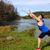## CodeNewbie Community is a community of 4,533 amazing newbies# Helpful math for machine learningTristan

# Introduction

• This series is going to be my attempt to build a very basic neural network in C++ from scratch. All the information from this series can be found in the book, C++ Neural Networks and Fuzzy Logic by Valluru B. Rao.

• On a more theoretical level of machine learning, according to the university of Toronto's machine learning and data mining class, found HERE , there are 4 sections of math that are helpful for machine learning.

1) Linear algebra: vector/matrix manipulations, properties